# Moderation

## The Conceptual Model

### Version 1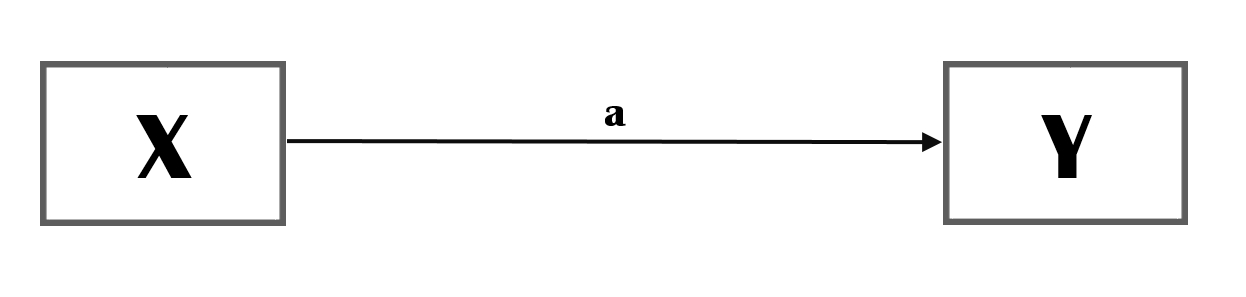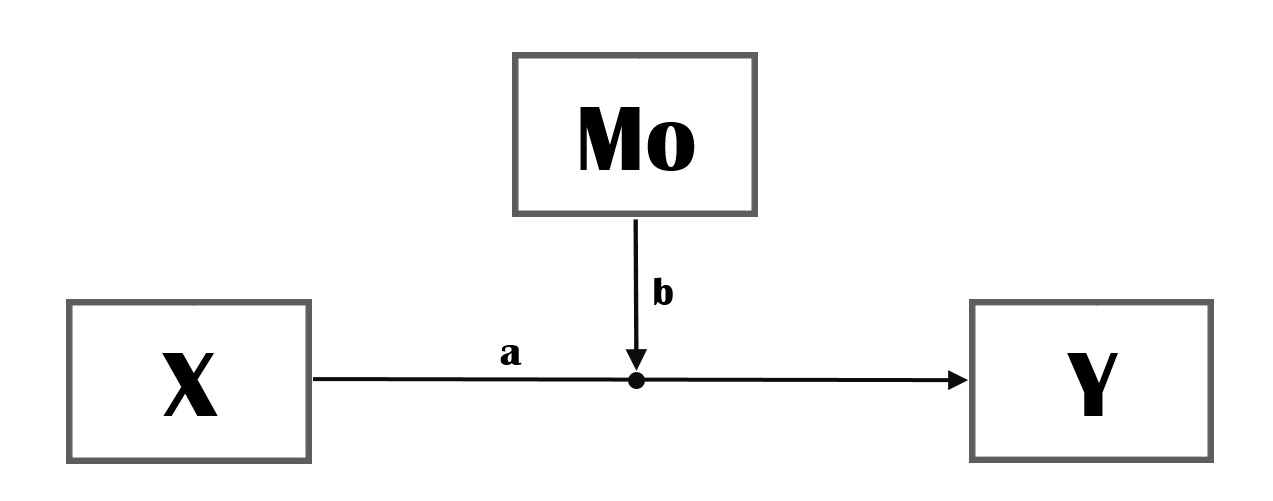Figure 1: Moderation

The moderator Mo moderates the strength of the impact of X on Y (i.e. path a).

### Version 2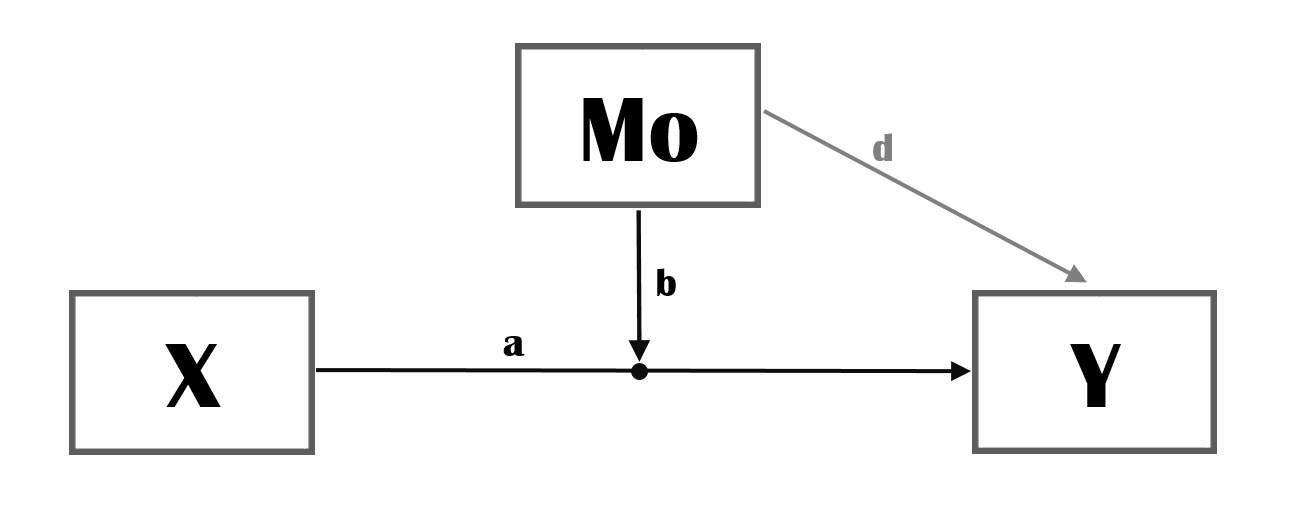Figure 2: Moderation (including direct effect of moderator)

In Version 2, the direct effect of the Moderator Mo on Y is also drawn. You may not have theorized an effect of Mo on Y and it thus not always necessary to draw this relationship but it is a good idea to consider whether this relationship may exist. When testing for the moderation effect b it is good practice for the direct of of the Moderator (path d) as it will facilitate the interpretation of the moderation effect.

### Version 3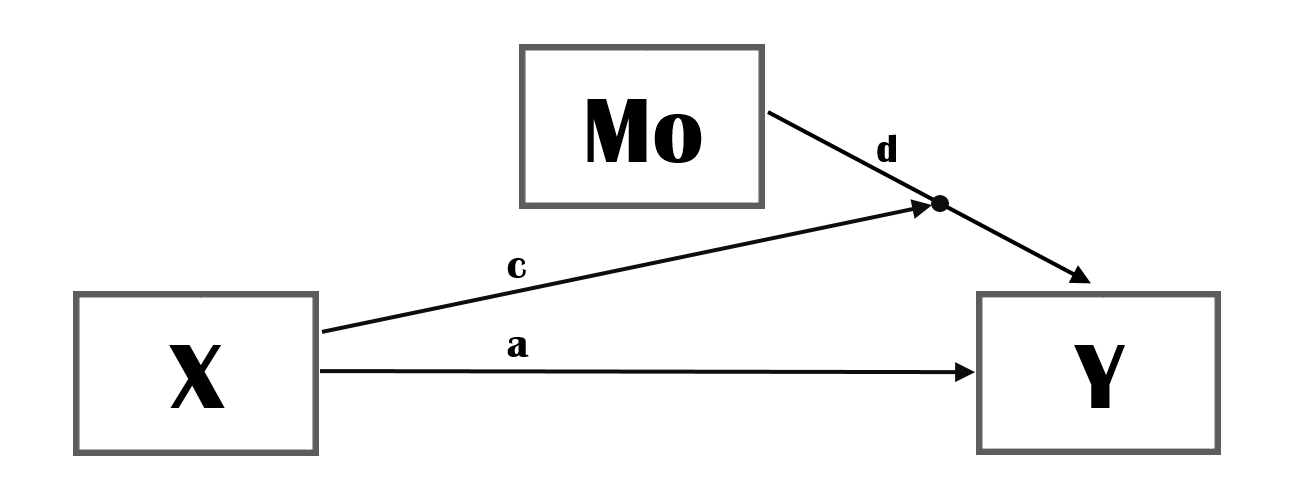Figure 3: Moderation (equivalent to version 2)

Version 2 and Version 3 are (mathematically) equivalent (structural) models. They will lead to exactly the same model fit. However, from a theoretical viewpoint they are different. (see also examples below).

### Version 4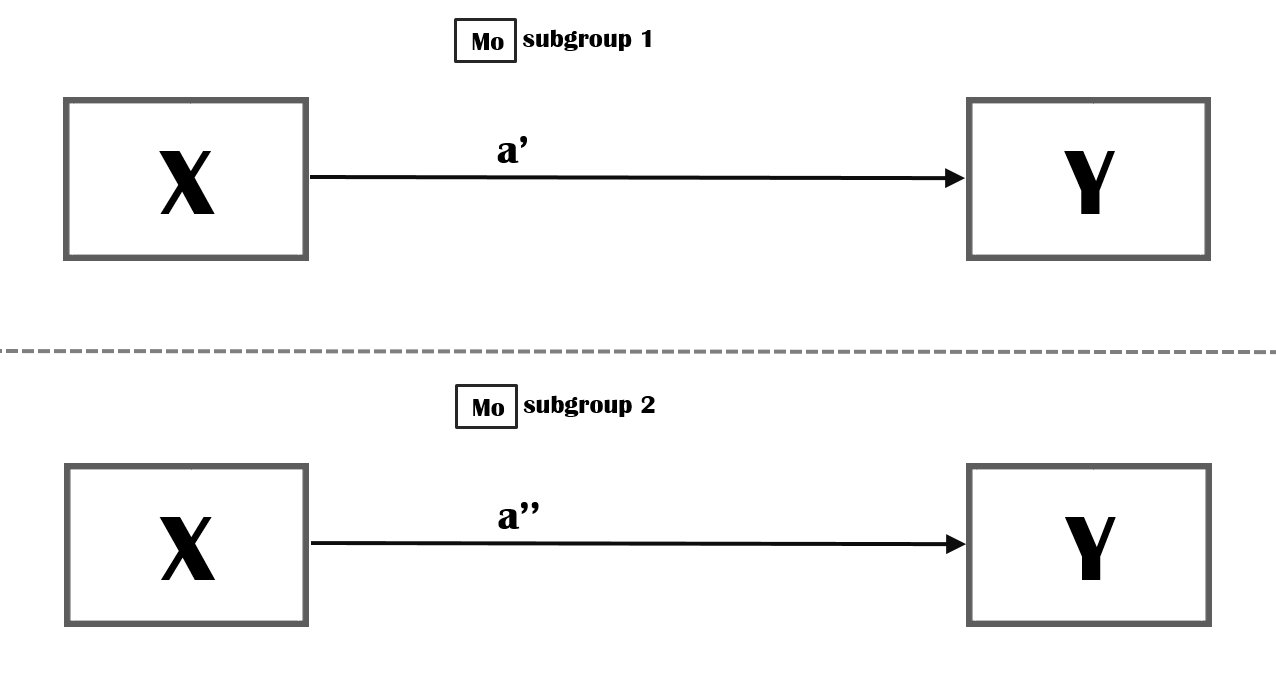Figure 4: Moderation (categorical Moderator)

If your Moderator is a nominal variable (e.g. gender) it may be more informative for a reader to graphically summarize the model for each category (e.g. men, women, other) separately. This may be especially useful if your model is more complex and includes more independent variables and or mediation variables and you expect more than one relationship to differ depending on gender.1

## Explanation

In a conceptual model, the concepts are normally placed in a rectangular. We have three concepts, X, Mo and Y.
We assume an association between X and Y. The strength of this relation may depend on Mo, the strength of this relation is conditional on the value of Mo. We also call this an interaction effect. If you want to graphically depict a moderation, it may be useful to show the model without the moderator as well but this is a bit a matter of taste. I have noticed that journal article reviewers and committees that evaluate grant proposals are not always familiar with a graphical depiction of an interaction effect. I have received (more than once!) the comment that my figure was presumably wrong because an arrow was pointing at a different arrow. I therefore now add a very small bullit on the arrow which, I hope, makes clear that it is by design that I point one arrow to another arrow.
It is not always necessary to label the paths but for this tutorial it will turn out to be handy. Normally, when there is no sign (or label) it is assumed that the path has a positive valence. It is, however, good practice to include the valence of the paths in your conceptual models.

If the relationship between X and Y is assumed to be non-linear, for example when the dependent variable is binary and you are planning to estimate logistic regression models, the interpretation of moderation/interaction effects is a lot more complicated. SEE STRUCTURAL EQUATIONS BELOW.

## Abstract hypotheses

See Version 1/2 above.

### positive main effect, positive interaction

• Hypo1: more X leads to more Y ($$a>0$$).
• Hypo2: The relationship between X and Y becomes stronger when Mo increases ($$b>0$$).

### positive main effect, negative interaction

• Hypo1: more X leads to more Y ($$a>0$$).
• Hypo2: The relationship between X and Y becomes weaker when Mo increases ($$b<0$$).

### negative main effect, positive interaction

• Hypo1: more X leads to less Y ($$a<0$$).
• Hypo2: The negative relationship between X and Y becomes weaker when Mo increases ($$b>0$$).

### negative main effect, negative interaction

• Hypo1: more X leads to less Y ($$a<0$$).
• Hypo2: The negative relationship between X and Y becomes stronger when Mo increases ($$b<0$$).

## Real life example

X is educational success Mo is age.
Y is health

• Hypo1: Educational success is (positively) related to a better health.
• Hypo2: The positive relationship between educational success and a better health is stronger for older persons.

Alternatively, one could also formulate just the interaction effect:

• Hypo3: The relationship between educational success and a better health is stronger for older persons.

For hypothesis 3 it is not necessary that there is also a main effect of education on health. Also, the direction of the interaction effect is left a bit implicit (presumably positive). But cannot be falsified when:

• there is no main effect and we observe an interaction effect (regardless of sign)
• there is a positive main effect and a positive interaction effect.
• there is a negative main effect and a negative interaction effect.

For this reason, I would prefer hypotheses 1 and 2 above the single hypothesis 3.

Please compare the following set of hypotheses:

• Hypo1: Educational success is (positively) related to a better health.
• Hypo2: Age is (negatively) related to a better health.
• Hypo3a: The positive relationship between educational success and a better health is stronger for older persons.

and:

• Hypo1: Educational success is (positively) related to a better health.
• Hypo2: Age is (negatively) related to a better health.
• Hypo3b: The negative relationship between age and a better health is weaker for higher educated persons.

Both sets will lead to the same structural equations. But do you think that for older persons education based inequality in health will be more pronounced or that for higher educated age based inequality in health will be less pronounced?

## Structural equations

• $Y= b_0 + b_1X + b_2Mo + b_3XMo Y= b_0 + (b_1 + b_3Mo)X + b_2Mo$

### Interpretation (linear model)

The interaction effect is the cross-partial derivative of Y with respect to X and M0:2

$\frac{\partial ^2(Y|X,Mo)}{\partial X \partial Mo} = \frac{\partial(b_1 + b_3Mo)}{ \partial Mo} = b_3$

Thus the interaction effect is $$b_3$$.

### Interpretation (non-linear (logit) model)

However, if we have a binary outcome variable our hypotheses are about the probability that Y is 1 ($$P(Y=1)$$). If we estimate the model with a logit function, this is:3

$$P(Y=1|X,Mo)= \frac{e^{(b_0 + b_1X + b_2Mo + b_3XMo)}} {1 + e^{(b_0 + b_1X + b_2Mo + b_3XMo)}} = \frac{1}{1 + e^{-(b_0 + b_1X + b_2Mo + b_3XMo)}}$$

let us define $$P(Y=1|X,Mo)$$ as $$F(Y)$$ (i.e. the logistic distribution function).

The interaction effect then becomes:

$\frac{\partial ^2 F(Y)}{\partial X \partial Mo} = \frac{\partial(f(Y)(b_1 + b_3Mo))}{ \partial Mo} = f(Y)b_3 + f'(Y)(b_1 + b_3Mo)(b_2 + b_3X),$ where $$f(Y)$$ is the derivative of the logistic distribution function (i.e. the logistic density function) and $$f'(Y)$$ the derivative of the density function with respect to Y.

For more background reading see Norton, Wang, and Ai (2004).

For now we have three take home messages!:

• even if $$b_3$$ is non significant you may have a significant interaction effect (namely: $$f'(Y)b_1b_2$$
• the strength, valence (and significance) of the interaction effect depends on the value of $$Y$$ (i.e. the covariates), $$b_1$$, $$b_2$$ and $$b_3$$.
• To make sense of interaction effects in nonlinear models, use (3D) plots of predicted values against values of X and Mo!

### Lavaan syntax

Following the syntax of the R package Lavaan

• Y~1
• Y~a*X
• Y~d*Mo
• Y~b*X:Mo

## Formal test of hypotheses

rm(list = ls())  #empty environment
require(haven)
nells <- read_dta("../static/NELLS panel nl v1_2.dta")  #change directory name to your working directory

Operationalize concepts. Please note that I mean center the covariates for ease of interpretation!

# We will use the data of wave 2.
nellsw2 <- nells[nells$w2cpanel == 1, ] # As an indicator of occupational success we will use income in wave 2. table(nellsw2$w2fa61, useNA = "always")
attributes(nellsw2$w2fa61) # recode (I will start newly created variables with cm from conceptual models) nellsw2$cm_income <- nellsw2$w2fa61 nellsw2$cm_income[nellsw2$cm_income == 1] <- 100 nellsw2$cm_income[nellsw2$cm_income == 2] <- 225 nellsw2$cm_income[nellsw2$cm_income == 3] <- 400 nellsw2$cm_income[nellsw2$cm_income == 4] <- 750 nellsw2$cm_income[nellsw2$cm_income == 5] <- 1250 nellsw2$cm_income[nellsw2$cm_income == 6] <- 1750 nellsw2$cm_income[nellsw2$cm_income == 7] <- 2250 nellsw2$cm_income[nellsw2$cm_income == 8] <- 2750 nellsw2$cm_income[nellsw2$cm_income == 9] <- 3250 nellsw2$cm_income[nellsw2$cm_income == 10] <- 3750 nellsw2$cm_income[nellsw2$cm_income == 11] <- 4250 nellsw2$cm_income[nellsw2$cm_income == 12] <- 4750 nellsw2$cm_income[nellsw2$cm_income == 13] <- 5250 nellsw2$cm_income[nellsw2$cm_income == 14] <- 5750 nellsw2$cm_income[nellsw2$cm_income == 15] <- 6500 nellsw2$cm_income[nellsw2$cm_income == 16] <- 7500 nellsw2$cm_income[nellsw2$cm_income == 17] <- NA # let us scale the variable a bit and translate into income per 1000euro nellsw2$cm_income <- nellsw2$cm_income/1000 # from household income to personal income attributes(nellsw2$w2fa62)
table(nellsw2$w2fa62, useNA = "always") nellsw2$cm_income_per <- nellsw2$w2fa62 nellsw2$cm_income_per[nellsw2$cm_income_per == 1] <- 0 nellsw2$cm_income_per[nellsw2$cm_income_per == 2] <- 10 nellsw2$cm_income_per[nellsw2$cm_income_per == 3] <- 20 nellsw2$cm_income_per[nellsw2$cm_income_per == 4] <- 30 nellsw2$cm_income_per[nellsw2$cm_income_per == 5] <- 40 nellsw2$cm_income_per[nellsw2$cm_income_per == 6] <- 50 nellsw2$cm_income_per[nellsw2$cm_income_per == 7] <- 60 nellsw2$cm_income_per[nellsw2$cm_income_per == 8] <- 70 nellsw2$cm_income_per[nellsw2$cm_income_per == 9] <- 80 nellsw2$cm_income_per[nellsw2$cm_income_per == 10] <- 90 nellsw2$cm_income_per[nellsw2$cm_income_per == 11] <- 100 nellsw2$cm_income_per[nellsw2$cm_income_per == 12] <- NA nellsw2$cm_income_ind <- nellsw2$cm_income * nellsw2$cm_income_per/100

# as an indicator of educational success we will use highest completed level of education in years.
# the rationale behind this coding this I will take the maximum for university as 16.5 (taking into
# account that some masters are 2 years and some 1 year) and subsequently subtract the years needed
# to obtain a university degree given the degree under consideration.

attributes(nellsw2$w2fa102) table(nellsw2$w2fa102, useNA = "always")
nellsw2$cm_education <- nellsw2$w2fa102
nellsw2$cm_education[nellsw2$w2fa102 == 1] <- 6
nellsw2$cm_education[nellsw2$w2fa102 == 2] <- 9
nellsw2$cm_education[nellsw2$w2fa102 == 3] <- 10
nellsw2$cm_education[nellsw2$w2fa102 == 4] <- 11
nellsw2$cm_education[nellsw2$w2fa102 == 5] <- 12
nellsw2$cm_education[nellsw2$w2fa102 == 6] <- 10
nellsw2$cm_education[nellsw2$w2fa102 == 7] <- 11
nellsw2$cm_education[nellsw2$w2fa102 == 8] <- 14
nellsw2$cm_education[nellsw2$w2fa102 == 9] <- 15
nellsw2$cm_education[nellsw2$w2fa102 == 10] <- 16.5
nellsw2$cm_education[nellsw2$w2fa102 == 11] <- 16.5
nellsw2$cm_education[nellsw2$w2fa102 == 12] <- 7
nellsw2$cm_education[nellsw2$w2fa102 == 13] <- 11
nellsw2$cm_education[nellsw2$w2fa102 == 14] <- 14.5
nellsw2$cm_education[nellsw2$w2fa102 == 15] <- 4

# mean centering
nellsw2$cm_education_c <- nellsw2$cm_education - mean(nellsw2$cm_education, na.rm = T) nellsw2$cm_age_c <- nellsw2$w1cage - mean(nellsw2$w1cage, na.rm = T)

# define a dichotemous moderator based on age.
nellsw2$cm_old_d <- ifelse(nellsw2$cm_age_c >= 0, 1, 0)

# as an indicator of health we will use subjective well being from 5 (excellent) to 1 (bad) thus we
# have to reverse code original variable
attributes(nellsw2$w2scf1) table(nellsw2$w2scf1, useNA = "always")
nellsw2$cm_health <- 6 - nellsw2$w2scf1
##
##    1    2    3    4    5    6    7    8    9   10   11   12   13   14   15   16   17 <NA>
##   55   78  103  204  338  326  282  272  276  205  133   62   48   22   22   29  374    0
## $label ##  " wat is het netto inkomen per maand van u en uw partner samen?/van u?/ " ## ##$format.stata
##  "%8.0g"
##
## $labels ## Minder dan ¤150 per maand ¤150 - ¤299 per maand ¤300 - ¤499 per maand ## 1 2 3 ## ¤500 - ¤999 per maand ¤1.000 - ¤1.499 per maand ¤1.500 - ¤1.999 per maand ## 4 5 6 ## ¤2.000 - ¤2.499 per maand ¤2.500 - ¤2.999 per maand ¤3.000 - ¤3.499 per maand ## 7 8 9 ## ¤3.500 - ¤3.999 per maand ¤4.000 - ¤4.499 per maand ¤4.500 - ¤4.999 per maand ## 10 11 12 ## ¤5.000 - ¤5.499 per maand ¤5.500 - ¤5.999 per maand ¤6.000 - ¤6.999 per maand ## 13 14 15 ## ¤7.000 of meer per maand weet niet, wil niet zeggen ## 16 17 ## ##$class
##  "haven_labelled" "vctrs_vctr"     "double"
##
## $label ##  " hoe groot is uw bijdrage in dit inkomen ongeveer? kunt u een percentage noemen " ## ##$format.stata
##  "%8.0g"
##
## $labels ## vrijwel geen bijdrage ongeveer 10% ongeveer 20% ongeveer 30% ## 1 2 3 4 ## ongeveer 40% ongeveer 50% ongeveer 60% ongeveer 70% ## 5 6 7 8 ## ongeveer 80% ongeveer 90% ongeveer 100% weet niet ## 9 10 11 12 ## ##$class
##  "haven_labelled" "vctrs_vctr"     "double"
##
##
##    1    2    3    4    5    6    7    8    9   10   11   12 <NA>
##  253   48   89  259  233  242  183  229  114   63  887  229    0
## $label ##  " wat is uw hoogst voltooide opleiding, dat wil zeggen waarvan u een diploma heef" ## ##$format.stata
##  "%8.0g"
##
## $labels ## lagere school ## 1 ## lbo, vmbo-kb\\bbl ## 2 ## mavo, vmbo-tl ## 3 ## havo ## 4 ## vwo\\gymnasium ## 5 ## mbo-kort (kmbo), primair leerlingwezen, bol\\bbl niveau 1 of ## 6 ## mbo-tussen\\lang (mbo), secundair\\tertiar leerlingwezen, bol\\ ## 7 ## hbo ## 8 ## universiteit (bachelor) ## 9 ## universiteit (master, doctoraal) ## 10 ## promotietraject ## 11 ## buitenlandse opleiding, niet goed in te delen, lager onderwi ## 12 ## buitenlandse opleiding, niet goed in te delen, middelbaar on ## 13 ## buitenlandse opleiding, niet goed in te delen, hoger onderwi ## 14 ## geen opleiding ## 15 ## ##$class
##  "haven_labelled" "vctrs_vctr"     "double"
##
##
##    1    2    3    4    5    6    7    8    9   10   11   12   13   14   15 <NA>
##  118  223  202  205  117  223  737  586   89  208   12    8   20   17   34   30
## $label ##  " wat vindt u, over het algemeen genomen, van uw gezondheid? " ## ##$format.stata
##  "%8.0g"
##
## $labels ## uitstekend zeer goed goed matig slecht ## 1 2 3 4 5 ## ##$class
##  "haven_labelled" "vctrs_vctr"     "double"
##
##
##    1    2    3    4    5 <NA>
##  438  853 1211  247   48   32

And test the model with Lavaan.

### Interaction variable approach

require(lavaan)

model <- "
#structural model
cm_health~ a*cm_education_c + d*cm_age_c + b*cm_education_c:cm_age_c
#intercepts
cm_health~1
cm_education_c ~1
cm_age_c~1

#residual variance
cm_health ~~ cm_health

#variances
cm_education_c ~~ cm_age_c
"

fit <- lavaan(model, data = nellsw2, auto.var = T, meanstructure = T)
summary(fit, standardized = TRUE)
## lavaan 0.6-7 ended normally after 31 iterations
##
##   Estimator                                         ML
##   Optimization method                           NLMINB
##   Number of free parameters                         11
##
##                                                   Used       Total
##   Number of observations                          2767        2829
##
## Model Test User Model:
##
##   Test statistic                               130.504
##   Degrees of freedom                                 3
##   P-value (Chi-square)                           0.000
##
## Parameter Estimates:
##
##   Standard errors                             Standard
##   Information                                 Expected
##   Information saturated (h1) model          Structured
##
## Regressions:
##                    Estimate  Std.Err  z-value  P(>|z|)   Std.lv  Std.all
##   cm_health ~
##     cm_edctn_c (a)    0.054    0.007    8.266    0.000    0.054    0.152
##     cm_age_c   (d)   -0.019    0.002  -10.558    0.000   -0.019   -0.194
##     cm_dct_:__ (b)    0.002    0.001    2.130    0.033    0.002    0.039
##
## Covariances:
##                     Estimate  Std.Err  z-value  P(>|z|)   Std.lv  Std.all
##   cm_education_c ~~
##     cm_age_c          -0.131    0.448   -0.292    0.770   -0.131   -0.006
##
## Intercepts:
##                    Estimate  Std.Err  z-value  P(>|z|)   Std.lv  Std.all
##    .cm_health         3.495    0.017  207.350    0.000    3.495    3.816
##     cm_education_c    0.002    0.049    0.039    0.969    0.002    0.001
##     cm_age_c         -0.065    0.174   -0.375    0.708   -0.065   -0.007
##     cm_dctn_c:cm__    0.000                               0.000    0.000
##
## Variances:
##                    Estimate  Std.Err  z-value  P(>|z|)   Std.lv  Std.all
##    .cm_health         0.786    0.021   37.195    0.000    0.786    0.937
##     cm_education_c    6.626    0.178   37.195    0.000    6.626    1.000
##     cm_age_c         83.937    2.257   37.195    0.000   83.937    1.000
##     cm_dctn_c:cm__  479.911   12.902   37.195    0.000  479.911    1.000

We observe that higher educated persons report higher SWB.
We observe that older persons report lower SWB.
We observe that the relationship between education and SWB is higher for older persons.

### multigroup approach

require(lavaan)
# no equality constraints across groups whatsoever.
model <- "
#structural model
cm_health~ c(a1,a0)*cm_education_c #I am giving the education effects specific names for each group
#intercepts
cm_health~1

#residual variance
cm_health ~~ cm_health

#test for difference
a1a0:=a1-a0
"

fit <- lavaan(model, data = nellsw2, auto.var = T, meanstructure = T, group = "cm_old_d")
summary(fit, standardized = TRUE)
## lavaan 0.6-7 ended normally after 15 iterations
##
##   Estimator                                         ML
##   Optimization method                           NLMINB
##   Number of free parameters                          6
##
##   Number of observations per group:               Used       Total
##     1                                             1530        1572
##     0                                             1237        1257
##
## Model Test User Model:
##
##   Test statistic                                 0.000
##   Degrees of freedom                                 0
##   Test statistic for each group:
##     1                                            0.000
##     0                                            0.000
##
## Parameter Estimates:
##
##   Standard errors                             Standard
##   Information                                 Expected
##   Information saturated (h1) model          Structured
##
##
## Group 1 :
##
## Regressions:
##                    Estimate  Std.Err  z-value  P(>|z|)   Std.lv  Std.all
##   cm_health ~
##     cm_dctn_c (a1)    0.066    0.008    7.895    0.000    0.066    0.198
##
## Intercepts:
##                    Estimate  Std.Err  z-value  P(>|z|)   Std.lv  Std.all
##    .cm_health         3.353    0.023  146.715    0.000    3.353    3.677
##
## Variances:
##                    Estimate  Std.Err  z-value  P(>|z|)   Std.lv  Std.all
##    .cm_health         0.799    0.029   27.659    0.000    0.799    0.961
##
##
## Group 2 :
##
## Regressions:
##                    Estimate  Std.Err  z-value  P(>|z|)   Std.lv  Std.all
##   cm_health ~
##     cm_dctn_c (a0)    0.038    0.011    3.549    0.000    0.038    0.100
##
## Intercepts:
##                    Estimate  Std.Err  z-value  P(>|z|)   Std.lv  Std.all
##    .cm_health         3.675    0.025  145.563    0.000    3.675    4.120
##
## Variances:
##                    Estimate  Std.Err  z-value  P(>|z|)   Std.lv  Std.all
##    .cm_health         0.788    0.032   24.870    0.000    0.788    0.990
##
## Defined Parameters:
##                    Estimate  Std.Err  z-value  P(>|z|)   Std.lv  Std.all
##     a1a0              0.028    0.014    2.083    0.037    0.028    0.097

We observe that the relationship between education and SWB is significantly higher for older persons.

1. You may also want to estimate the model separately for subgroups because you expect different (error)variances in your dependent variable across subgroups.↩︎

2. Assuming continuous variables X and Mo.↩︎

3. The same logic applies to other nonlinear models of course.↩︎